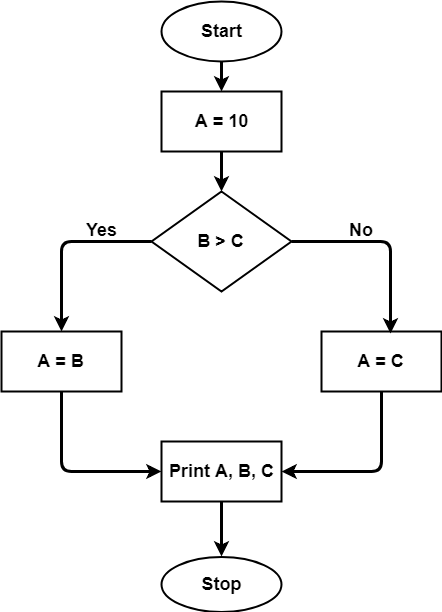# Cyclomatic Complexity

• Difficulty Level : Easy
• Last Updated : 27 Jul, 2022

Cyclomatic complexity of a code section is the quantitative measure of the number of linearly independent paths in it. It is a software metric used to indicate the complexity of a program. It is computed using the Control Flow Graph of the program. The nodes in the graph indicate the smallest group of commands of a program, and a directed edge in it connects the two nodes i.e. if second command might immediately follow the first command.

For example, if source code contains no control flow statement then its cyclomatic complexity will be 1 and source code contains a single path in it. Similarly, if the source code contains one if condition then cyclomatic complexity will be 2 because there will be two paths one for true and the other for false.

Mathematically, for a structured program, the directed graph inside control flow is the edge joining two basic blocks of the program as control may pass from first to second.
So, cyclomatic complexity M would be defined as,

M = E – N + 2P

where,
E = the number of edges in the control flow graph
N = the number of nodes in the control flow graph
P = the number of connected components

Steps that should be followed in calculating cyclomatic complexity and test cases design are:

• Construction of graph with nodes and edges from code.
• Identification of independent paths.
• Cyclomatic Complexity Calculation
• Design of Test Cases

Let a section of code as such:

```A = 10
IF B > C THEN
A = B
ELSE
A = C
ENDIF
Print A
Print B
Print C```

Control Flow Graph of above codeThe cyclomatic complexity calculated for above code will be from control flow graph. The graph shows seven shapes(nodes), seven lines(edges), hence cyclomatic complexity is 7-7+2 = 2.

Use of Cyclomatic Complexity:

• Determining the independent path executions thus proven to be very helpful for Developers and Testers.
• It can make sure that every path have been tested at least once.
• Thus help to focus more on uncovered paths.
• Code coverage can be improved.
• Risk associated with program can be evaluated.
• These metrics being used earlier in the program helps in reducing the risks.

Advantages of Cyclomatic Complexity:.

• It can be used as a quality metric, gives relative complexity of various designs.
• It is able to compute faster than the Halstead’s metrics.
• It is used to measure the minimum effort and best areas of concentration for testing.
• It is able to guide the testing process.
• It is easy to apply.

Disadvantages of Cyclomatic Complexity:

• It is the measure of the programs’s control complexity and not the data complexity.
• In this, nested conditional structures are harder to understand than non-nested structures.
• In case of simple comparisons and decision structures, it may give a misleading figure.
My Personal Notes arrow_drop_up